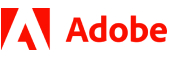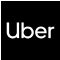New update is available. Click here to update.
Topics

# Kevin and the tower of coins

Moderate0/80
Average time to solve is 25m+2 more companies

## Problem statement

Kevin has ‘N’ coins. Each coin has a specific width and diameter. Kevin wants to build a tower using these coins such that each coin in the tower has strictly less width and diameter as compared to all coins placed below this coin.

You have to find the maximum height of the tower that Kevin can build by using these coins.

Note:

``````The height of the tower is calculated by adding the width of all the coins used in the formation of this tower.
``````
Detailed explanation ( Input/output format, Notes, Images )
Constraints:
``````1 <= T <= 50
1 <= N <= 10^4
1 <= ARR[i] and ARR[i] <= 10^5

Time limit: 1 sec
``````
Sample Input 1:
``````2
2
4 5
2 4
4
1 1
2 2
3 3
4 4
``````
Sample Output 1:
``````2
4
``````
Explanation of sample input 1:
``````In the first test case, the tower formed will have the 2nd coin at the lowest level and the 1st coin on top of it.

In the second test case, the 4th coin will be placed at the bottom of the tower, on top of that is 3rd and on whose top is 2nd and at the topmost level is 1st coin.
``````
Sample Input 2:
``````2
1
5 4
3
4 2
6 1
1 10
``````
Sample Output 2:
``````1
1
``````
Explanation for sample input 2:
``````In the first test case, the tower will only be formed by a single given coin.

In the second test case, the tower will only be formed by using a single coin.
``````Console